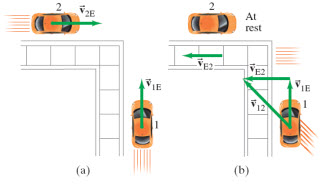# Problem: Two cars approach a street corner at right angles to each other (as show in the figure). Car 1 travels at 35 km/h and car 2 at 45 km/h. Assume that car 1 is moving in positive direction along axis y and car 2 is moving in positive direction along axis x. What is the relative velocity of car 1 as seen by car 2?What is the velocity of car 2 relative to car 1?

⚠️Our tutors found the solution shown to be helpful for the problem you're searching for. We don't have the exact solution yet.

###### Problem Details
Two cars approach a street corner at right angles to each other (as show in the figure). Car 1 travels at 35 km/h and car 2 at 45 km/h. Assume that car 1 is moving in positive direction along axis y and car 2 is moving in positive direction along axis x.What is the relative velocity of car 1 as seen by car 2?

What is the velocity of car 2 relative to car 1?